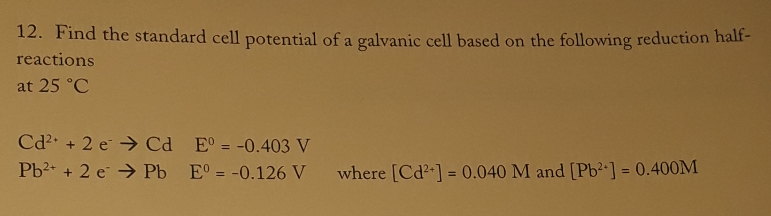# 12. Find the standard cell potential of a galvanic cell based on the following reduction half-reactions at 25 degrees C. Cd^2+ + 2e^- → Cd E° = -0.403 V Pb^2+ + 2e^- → Pb E° = -0.126 V where [Cd^2+] = 0.040 M and [Pb^2+] = 0.400M